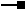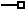# IGeometryDefEdit Interface

## Members

Name DescriptionAvgNumPoints The estimated average number of points per feature.AvgNumPoints The estimated average number of points per feature.GeometryType The geometry type.GeometryType The enumerated geometry type.GridCount The number of spatial index grids.GridCount The number of spatial index grids.GridSize The size of a spatial index grid.GridSize The size of a spatial index grid.HasM Indicates if the feature class will support M values.HasM Indicates if the feature class has measure (M) values.HasZ Indicates if the feature class will support Z values.HasZ Indicates if the featureClass has Z values.SpatialReference The spatial reference of the dataset.SpatialReference The spatial reference for the dataset.

### IGeometryDefEdit.AvgNumPoints Property

The estimated average number of points per feature.

``````Public WriteOnly Property AvgNumPoints_2
``````
``````public void AvgNumPoints_2 {set;}
``````

### IGeometryDefEdit.AvgNumPoints Property

The estimated average number of points per feature.

``````Public WriteOnly Property AvgNumPoints_2
``````
``````public void AvgNumPoints_2 {set;}
``````

### IGeometryDefEdit.GeometryType Property

The geometry type.

``````Public WriteOnly Property GeometryType_2
``````
``````public void GeometryType_2 {set;}
``````

#### Remarks

The following are valid Geometry types for a new feature class (from esriGeometryType):

esriGeometryPoint

esriGeometryMultipoint

esriGeometryPolyline

esriGeometryPolygon

esriGeometryMultiPatch

### IGeometryDefEdit.GeometryType Property

The geometry type.

``````Public WriteOnly Property GeometryType_2
``````
``````public void GeometryType_2 {set;}
``````

#### Remarks

The following are valid Geometry types for a new feature class (from esriGeometryType):

esriGeometryPoint

esriGeometryMultipoint

esriGeometryPolyline

esriGeometryPolygon

esriGeometryMultiPatch

### IGeometryDefEdit.GridCount Property

The number of spatial index grids.

``````Public WriteOnly Property GridCount_2
``````
``````public void GridCount_2 {set;}
``````

#### Remarks

GridCount specifies the number of spatial index grids. Personal Geodatabase feature classes only support one spatial index. Any additional indexes will be ignored. File and ArcSDE Geodatabase feature classes support up to 3 spatial indexes. Each additional index must be larger than the previous index.

### IGeometryDefEdit.GridCount Property

The number of spatial index grids.

``````Public WriteOnly Property GridCount_2
``````
``````public void GridCount_2 {set;}
``````

#### Remarks

GridCount specifies the number of spatial index grids. Personal Geodatabase feature classes only support one spatial index. Any additional indexes will be ignored. File and ArcSDE Geodatabase feature classes support up to 3 spatial indexes. Each additional index must be larger than the previous index.

### IGeometryDefEdit.GridSize Property

The size of a spatial index grid.

``````Public Sub set_GridSize ( _
ByVal Index As Integer, _
ByVal A_2 As Double _
)
``````
``````public void set_GridSize (
int Index,
double A_2
);
``````

#### Remarks

GridSize specifies the size of the spatial index. Personal Geodatabases feature classes support one spatial index while File and ArcSDE Geodatabase feature classes support up to 3 spatial indexes. Note that each additional index must be larger than the previous index. The GridSize index is zero based with a maximum index of 2.

If an R-Tree index is being used as a spatial index (e.g. Oracle Spatial, Informix, PostgreSQL), this property should be set to 0.

### IGeometryDefEdit.GridSize Property

The size of a spatial index grid.

``````Public Sub set_GridSize ( _
ByVal Index As Integer, _
ByVal A_2 As Double _
)
``````
``````public void set_GridSize (
int Index,
double A_2
);
``````

#### Remarks

GridSize specifies the size of the spatial index. Personal Geodatabases feature classes support one spatial index while File and ArcSDE Geodatabase feature classes support up to 3 spatial indexes. Note that each additional index must be larger than the previous index. The GridSize index is zero based with a maximum index of 2.

If an R-Tree index is being used as a spatial index (e.g. Oracle Spatial, Informix, PostgreSQL), this property should be set to 0.

### IGeometryDefEdit.HasM Property

Indicates if the feature class will support M values.

``````Public WriteOnly Property HasM_2
``````
``````public void HasM_2 {set;}
``````

### IGeometryDefEdit.HasM Property

Indicates if the feature class will support M values.

``````Public WriteOnly Property HasM_2
``````
``````public void HasM_2 {set;}
``````

### IGeometryDefEdit.HasZ Property

Indicates if the feature class will support Z values.

``````Public WriteOnly Property HasZ_2
``````
``````public void HasZ_2 {set;}
``````

### IGeometryDefEdit.HasZ Property

Indicates if the feature class will support Z values.

``````Public WriteOnly Property HasZ_2
``````
``````public void HasZ_2 {set;}
``````

### IGeometryDefEdit.SpatialReference Property

The spatial reference of the dataset.

``````Public WriteOnly Property SpatialReference_2
``````
``````public void SpatialReference_2 {set;}
``````

### IGeometryDefEdit.SpatialReference Property

The spatial reference of the dataset.

``````Public WriteOnly Property SpatialReference_2
``````
``````public void SpatialReference_2 {set;}
``````

## Inherited Interfaces

Interfaces Description

## Classes that implement IGeometryDefEdit

Classes Description
GeometryDef Esri Geometry Definition object.

## Remarks

The IGeometryDefEditinterface is used when creating a GeometryDefobject. You would normally use this interface when defining a new feature class. You cannot use IGeometryDefEditto modify an existing GeometryDefwhich is attached to a feature class; the same restrictions apply when using the standard ArcGIS user interface. For example, you cannot change the spatial reference of an existing feature class.

The following are valid Geometry types for a new feature class (from esriGeometryType):

esriGeometryPoint

esriGeometryMultipoint

esriGeometryPolyline

esriGeometryPolygon

esriGeometryMultiPatch

The SpatialReference property should always be set when creating a new GeometryDef. If a GeometryDef without a spatial reference is used to create a stand-alone feature class, an error will occur.

If a feature class is created in a feature dataset, and the SpatialReference property does not match the spatial reference of the feature dataset, the property will be modified to match that of the feature dataset.

``````The code below shows how to create a geometry field, assuming a spatial reference has already been created:

// Create the GeometryDef and set its spatial reference and geometry type.

IGeometryDef geometryDef = new GeometryDefClass();

IGeometryDefEdit geometryDefEdit = (IGeometryDefEdit)geometryDef;

geometryDefEdit.SpatialReference_2 = spatialReference;

geometryDefEdit.GeometryType_2 = esriGeometryType.esriGeometryPolyline;

// Set the grid count to 1 and the grid size to 0 to allow ArcGIS to

// determine a valid grid size.

geometryDefEdit.GridCount_2 = 1;

geometryDefEdit.set_GridSize(0, 0);

// Create a geometry field and assign the GeometryDef to it.

IField field = new FieldClass();

IFieldEdit fieldEdit = (IFieldEdit)field;

fieldEdit.Name_2 = "SHAPE";

fieldEdit.Type_2 = esriFieldType.esriFieldTypeGeometry;

fieldEdit.GeometryDef_2 = geometryDef;
``````
``````The code below shows how to create a geometry field, assuming a spatial reference has already been created:

' Create the GeometryDef and set its spatial reference and geometry type.

Dim geometryDef As IGeometryDef = New GeometryDefClass()

Dim geometryDefEdit As IGeometryDefEdit = CType(geometryDef, IGeometryDefEdit)

geometryDefEdit.SpatialReference_2 = spatialReference

geometryDefEdit.GeometryType_2 = esriGeometryType.esriGeometryPolyline

' Set the grid count to 1 and the grid size to 0 to allow ArcGIS to

' determine a valid grid size.

geometryDefEdit.GridCount_2 = 1

geometryDefEdit.GridSize_2(0) = 0

' Create a geometry field and assign the GeometryDef to it.

Dim field As IField = New FieldClass()

Dim fieldEdit As IFieldEdit = CType(field, IFieldEdit)

fieldEdit.Name_2 = "SHAPE"

fieldEdit.Type_2 = esriFieldType.esriFieldTypeGeometry

fieldEdit.GeometryDef_2 = geometryDef
``````